# Selina Solutions Concise Mathematics Class 6 Chapter 14: Fractions Exercise 14(E)

Selina Solutions Concise Mathematics Class 6 Chapter14: Fractions Exercise 14(E) provides students a clear cut picture of the concepts covered under this exercise, along with suitable examples. The solutions are designed by faculty at BYJU’S, and help them to achieve high marks in the final examinations. Students who find difficulty in solving the exercise wise problems are advised to refer to Selina Solutions PDF, which are available online and offline at any time. To obtain more details about the concepts, students can download the Selina Solutions Concise Mathematics Class 6 Chapter 14 Fractions Exercise 14(E) PDF free, from the links which are given below.

## Selina Solutions Concise Mathematics Class 6 Chapter 14 Fractions Exercise 14(E) Download PDF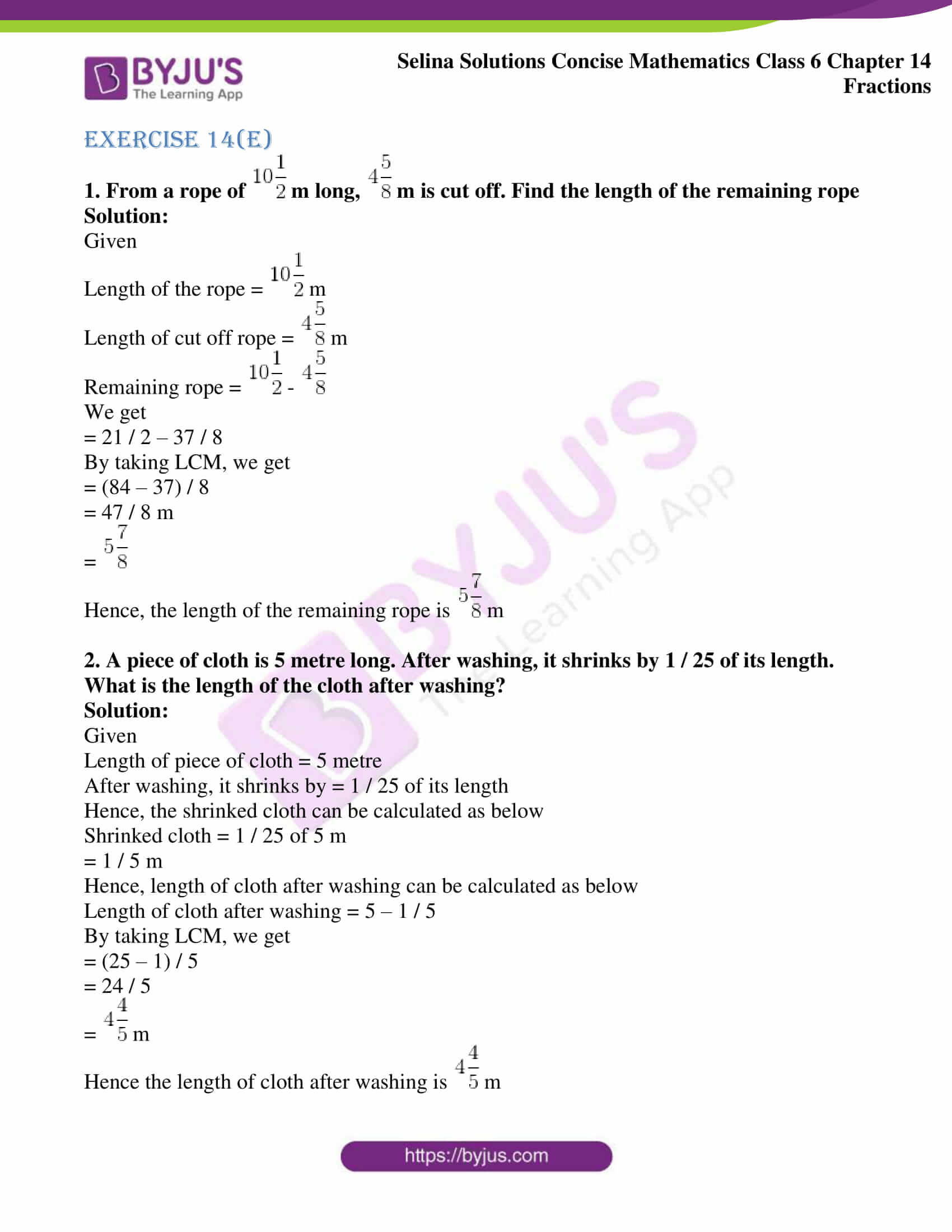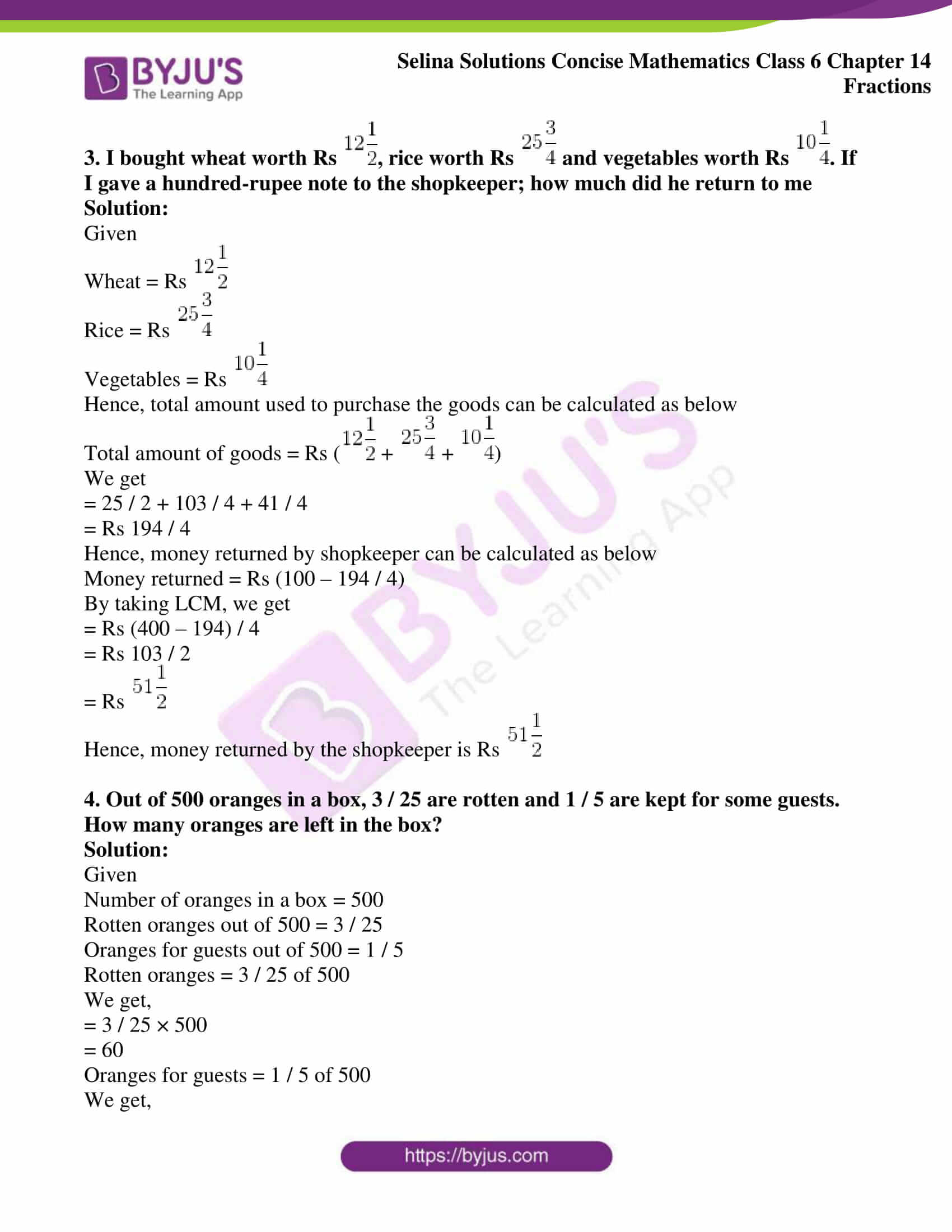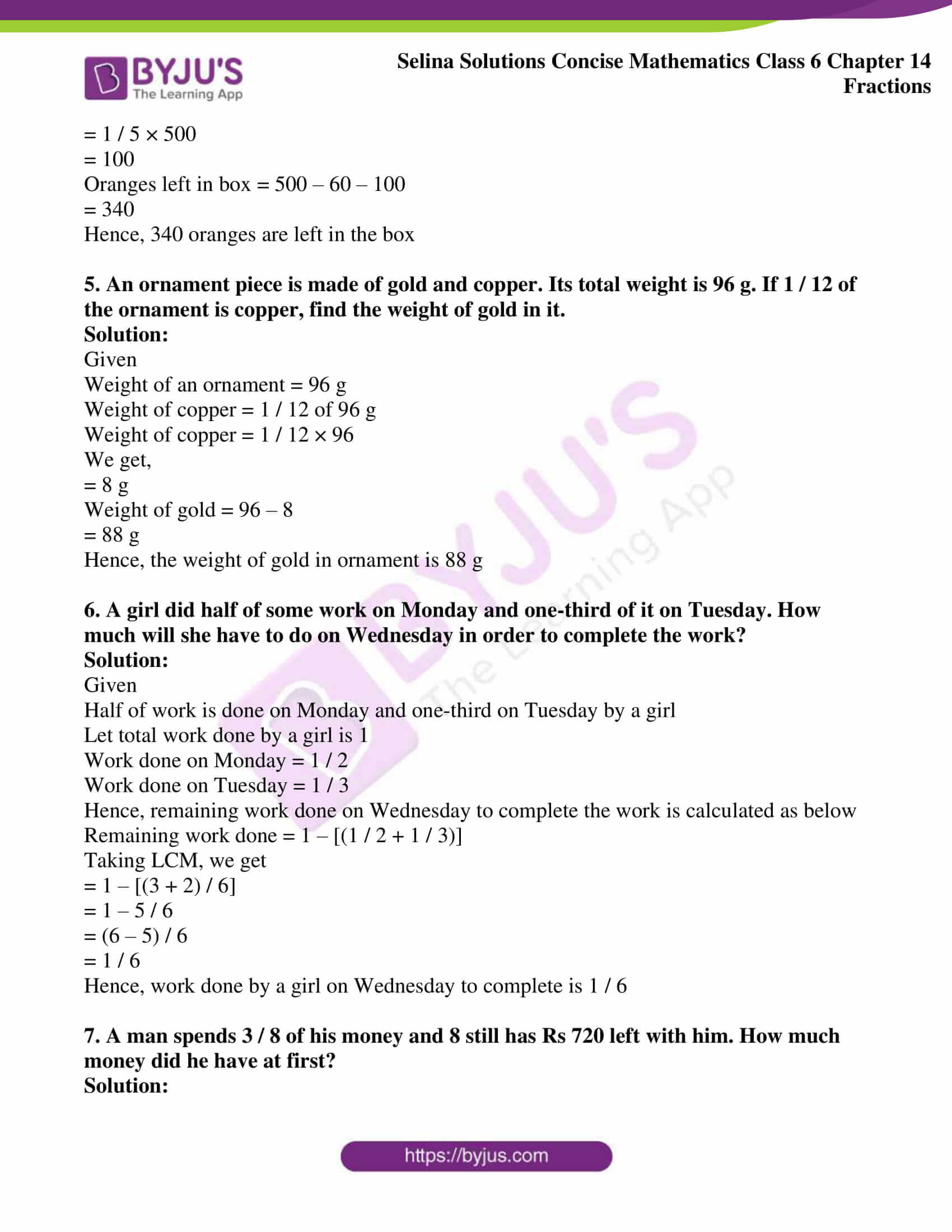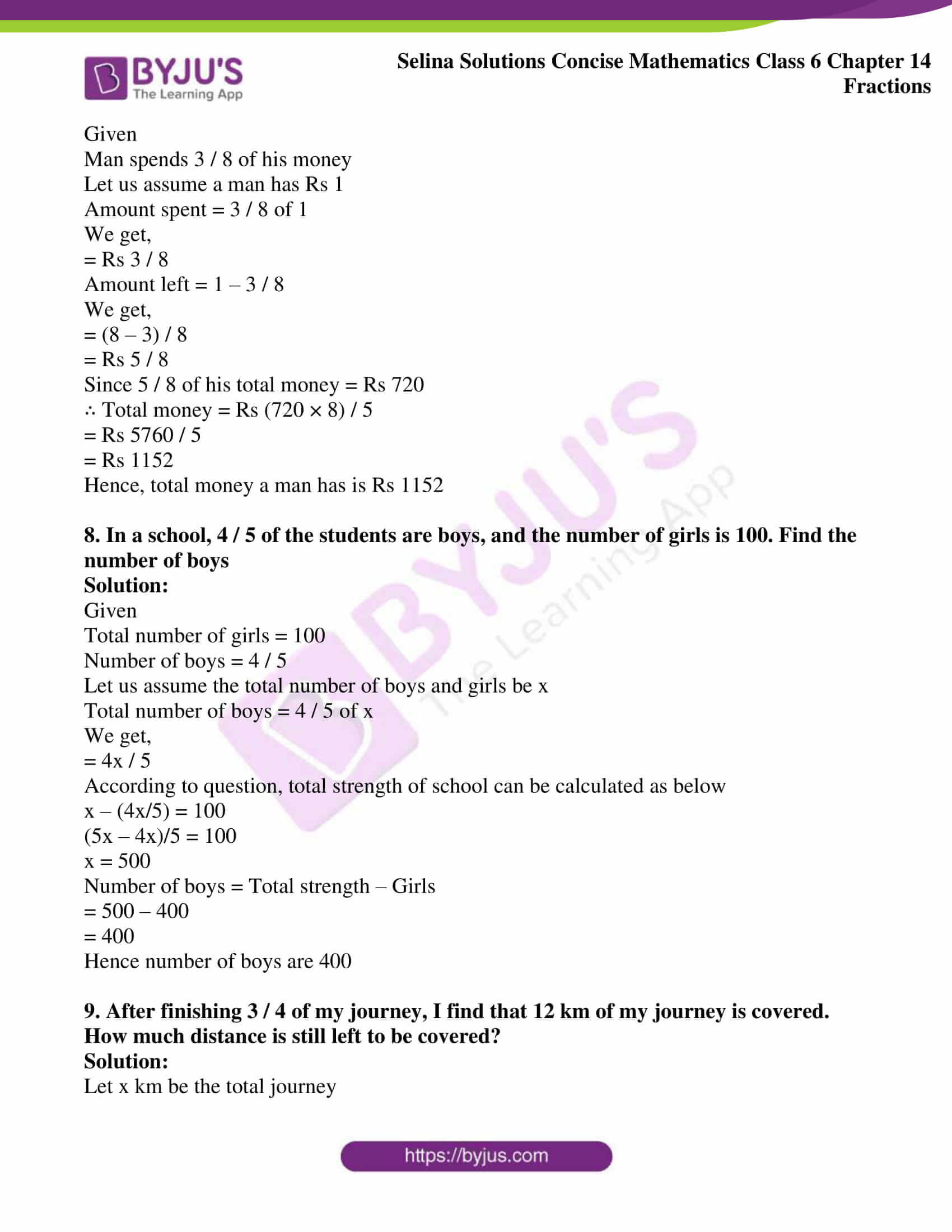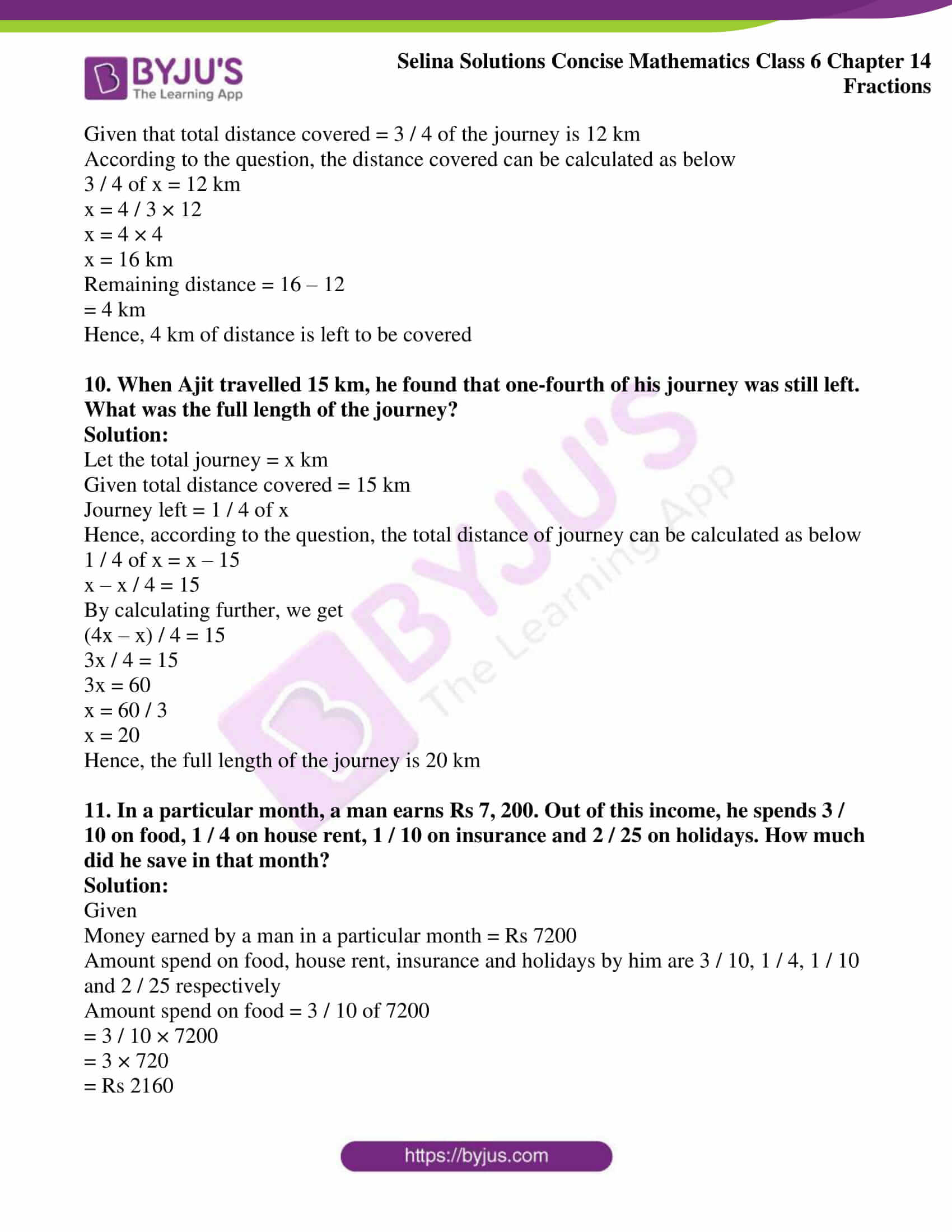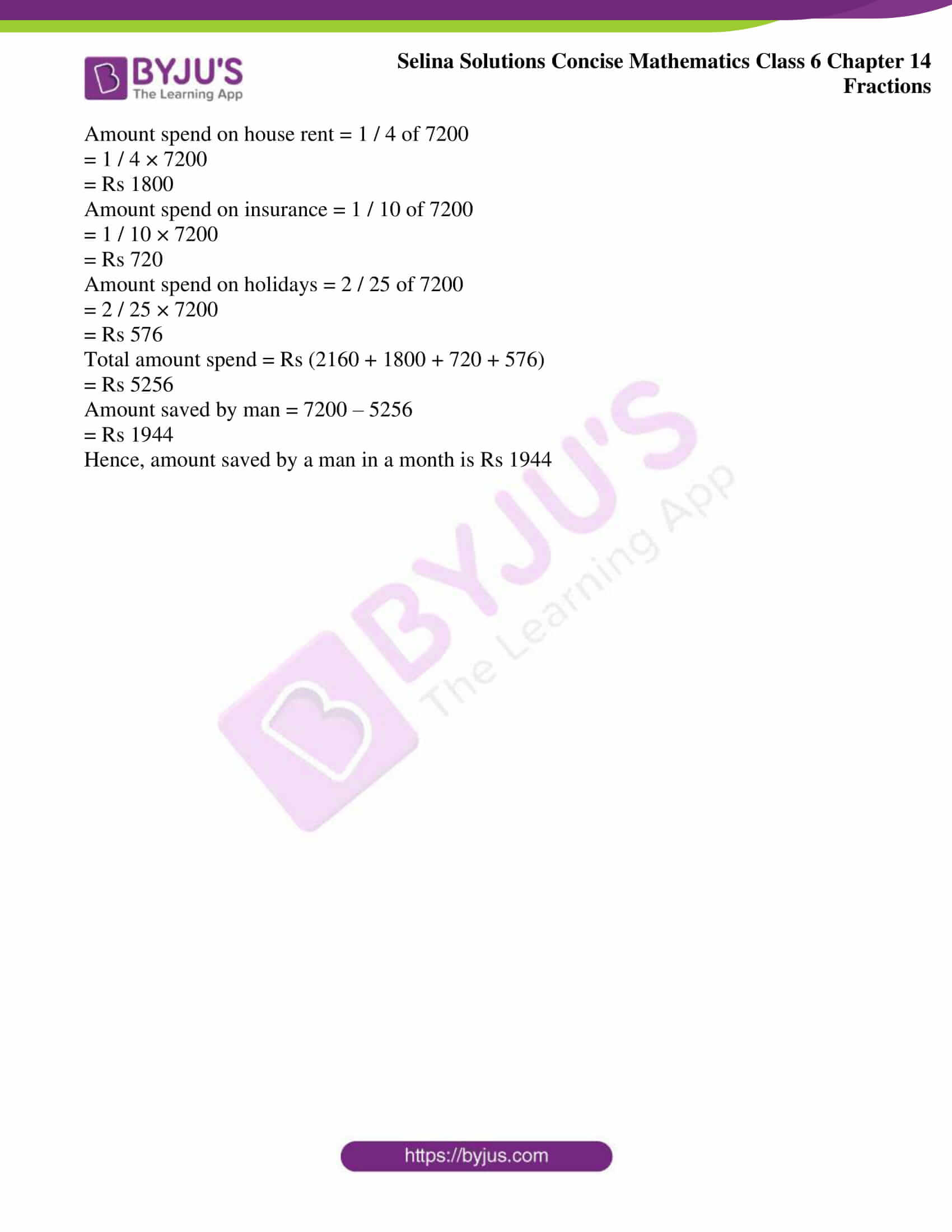### Access other exercises of Selina Solutions Concise Mathematics Class 6 Chapter 14: Fractions

Exercise 14(A) Solutions

Exercise 14(B) Solutions

Exercise 14(C) Solutions

Exercise 14(D) Solutions

### Access Selina Solutions Concise Mathematics Class 6 Chapter 14: Fractions Exercise 14(E)

Exercise 14(E)

1. From a rope of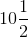m long,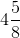m is cut off. Find the length of the remaining rope

Solution:

Given

Length of the rope =m

Length of cut off rope =m

Remaining rope =We get

= 21 / 2 – 37 / 8

By taking LCM, we get

= (84 – 37) / 8

= 47 / 8 m

=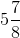Hence, the length of the remaining rope ism

2. A piece of cloth is 5 metre long. After washing, it shrinks by 1 / 25 of its length. What is the length of the cloth after washing?

Solution:

Given

Length of piece of cloth = 5 metre

After washing, it shrinks by = 1 / 25 of its length

Hence, the shrinked cloth can be calculated as below

Shrinked cloth = 1 / 25 of 5 m

= 1 / 5 m

Hence, length of cloth after washing can be calculated as below

Length of cloth after washing = 5 – 1 / 5

By taking LCM, we get

= (25 – 1) / 5

= 24 / 5

=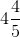m

Hence the length of cloth after washing ism

3. I bought wheat worth Rs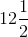, rice worth Rs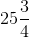and vegetables worth Rs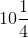. If I gave a hundred-rupee note to the shopkeeper; how much did he return to me

Solution:

Given

Wheat = RsRice = RsVegetables = RsHence, total amount used to purchase the goods can be calculated as below

Total amount of goods = Rs (++)

We get

= 25 / 2 + 103 / 4 + 41 / 4

= Rs 194 / 4

Hence, money returned by shopkeeper can be calculated as below

Money returned = Rs (100 – 194 / 4)

By taking LCM, we get

= Rs (400 – 194) / 4

= Rs 103 / 2

= Rs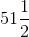Hence, money returned by the shopkeeper is Rs4. Out of 500 oranges in a box, 3 / 25 are rotten and 1 / 5 are kept for some guests. How many oranges are left in the box?

Solution:

Given

Number of oranges in a box = 500

Rotten oranges out of 500 = 3 / 25

Oranges for guests out of 500 = 1 / 5

Rotten oranges = 3 / 25 of 500

We get,

= 3 / 25 × 500

= 60

Oranges for guests = 1 / 5 of 500

We get,

= 1 / 5 × 500

= 100

Oranges left in box = 500 – 60 – 100

= 340

Hence, 340 oranges are left in the box

5. An ornament piece is made of gold and copper. Its total weight is 96 g. If 1 / 12 of the ornament is copper, find the weight of gold in it.

Solution:

Given

Weight of an ornament = 96 g

Weight of copper = 1 / 12 of 96 g

Weight of copper = 1 / 12 × 96

We get,

= 8 g

Weight of gold = 96 – 8

= 88 g

Hence, the weight of gold in ornament is 88 g

6. A girl did half of some work on Monday and one-third of it on Tuesday. How much will she have to do on Wednesday in order to complete the work?

Solution:

Given

Half of work is done on Monday and one-third on Tuesday by a girl

Let total work done by a girl is 1

Work done on Monday = 1 / 2

Work done on Tuesday = 1 / 3

Hence, remaining work done on Wednesday to complete the work is calculated as below

Remaining work done = 1 – [(1 / 2 + 1 / 3)]

Taking LCM, we get

= 1 – [(3 + 2) / 6]

= 1 – 5 / 6

= (6 – 5) / 6

= 1 / 6

Hence, work done by a girl on Wednesday to complete is 1 / 6

7. A man spends 3 / 8 of his money and 8 still has Rs 720 left with him. How much money did he have at first?

Solution:

Given

Man spends 3 / 8 of his money

Let us assume a man has Rs 1

Amount spent = 3 / 8 of 1

We get,

= Rs 3 / 8

Amount left = 1 – 3 / 8

We get,

= (8 – 3) / 8

= Rs 5 / 8

Since 5 / 8 of his total money = Rs 720

∴ Total money = Rs (720 × 8) / 5

= Rs 5760 / 5

= Rs 1152

Hence, total money a man has is Rs 1152

8. In a school, 4 / 5 of the students are boys, and the number of girls is 100. Find the number of boys

Solution:

Given

Total number of girls = 100

Number of boys = 4 / 5

Let us assume the total number of boys and girls be x

Total number of boys = 4 / 5 of x

We get,

= 4x / 5

According to question, total strength of school can be calculated as below

x – (4x/5) = 100

(5x – 4x)/5 = 100

x = 500

Number of boys = Total strength – Girls

= 500 – 400

= 400

Hence number of boys are 400

9. After finishing 3 / 4 of my journey, I find that 12 km of my journey is covered. How much distance is still left to be covered?

Solution:

Let x km be the total journey

Given that total distance covered = 3 / 4 of the journey is 12 km

According to the question, the distance covered can be calculated as below

3 / 4 of x = 12 km

x = 4 / 3 × 12

x = 4 × 4

x = 16 km

Remaining distance = 16 – 12

= 4 km

Hence, 4 km of distance is left to be covered

10. When Ajit travelled 15 km, he found that one-fourth of his journey was still left. What was the full length of the journey?

Solution:

Let the total journey = x km

Given total distance covered = 15 km

Journey left = 1 / 4 of x

Hence, according to the question, the total distance of journey can be calculated as below

1 / 4 of x = x – 15

x – x / 4 = 15

By calculating further, we get

(4x – x) / 4 = 15

3x / 4 = 15

3x = 60

x = 60 / 3

x = 20

Hence, the full length of the journey is 20 km

11. In a particular month, a man earns Rs 7, 200. Out of this income, he spends 3 / 10 on food, 1 / 4 on house rent, 1 / 10 on insurance and 2 / 25 on holidays. How much did he save in that month?

Solution:

Given

Money earned by a man in a particular month = Rs 7200

Amount spend on food, house rent, insurance and holidays by him are 3 / 10, 1 / 4, 1 / 10 and 2 / 25 respectively

Amount spend on food = 3 / 10 of 7200

= 3 / 10 × 7200

= 3 × 720

= Rs 2160

Amount spend on house rent = 1 / 4 of 7200

= 1 / 4 × 7200

= Rs 1800

Amount spend on insurance = 1 / 10 of 7200

= 1 / 10 × 7200

= Rs 720

Amount spend on holidays = 2 / 25 of 7200

= 2 / 25 × 7200

= Rs 576

Total amount spend = Rs (2160 + 1800 + 720 + 576)

= Rs 5256

Amount saved by man = 7200 – 5256

= Rs 1944

Hence, amount saved by a man in a month is Rs 1944